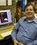Notice: This is an archived and unmaintained page. For current information, please browse astrobiology.nasa.gov.NAI

## 2011 Annual Science Report

### Task 2.1.1 Master Atmospheric Chemistry Simulation

##### Project Summary

The development of a master atmospheric model is nearing completion. Based on an older Titan model, the current model has been updated this year to include a treatment of chemical equilibrium, a description of aerosols, and a numerical model for condensation on and sublimation of atmospheric organic molecules from aerosol particles. To allow a global simulation of Titan atmospheric organic chemistry, the computer model is being recoded to support parallel processing.

4 Institutions
3 Teams
0 Publications
0 Field Sites
Field Sites

#### Project Progress

Participant Karen Willacy and Principal Investigator Mark Allen are developing a chemical/transport code that can model the atmosphere of Titan from the surface of the moon to the top of the atmosphere (1200 km). New features in this model needed for the Titan simulation include a treatment of chemical equilibrium (appropriate for high densities under certain circumstances), a description of the aerosols, and volatile condensation on and sublimation from aerosol particles.

The Caltech/JPL KINETICS model uses rate equations to simulate chemistry in a planetary atmosphere. Detailed chemical balance can be simulated by defining reverse reactions to complement the forward reactions so that under appropriate conditions thermochemical equilibrium can be computed. To do this the rate expression must be known for at least one of the forward or reverse reactions. The rate constant for the other reaction can be calculated in KINETICS by (equation 1)

K_eq=k_f/k_r

where Keq is the equilibrium constant, kf is the rate constant for the forward reaction, and kr is the rate constant for the reverse reaction.

KINETICS has been modified to include input data that are fits to the Gibbs free energy for each species (where these are available). These data are read in by KINETICS and used to calculate the reverse reaction rate constants. In this way each reaction is able to proceed both forwards and backwards, and chemical equilibrium is reached under the appropriate conditions of high densities and temperatures.

Work has proceeded on developing a treatment of the condensation and sublimation of ices onto existing aerosol particles. Condensation will occur if the partial pressure of a gas exceeds its saturation pressure. In interstellar chemical models (a guide for the Titan model) this is included in the kinetic chemical equations as (equation 2)

kcondense=Sx σ vx

where kcondense is the condensation rate, Sx is the sticking coefficient, σ is the cross-section of the grain and Vx is the gas phase velocity of the condensate.
The sublimation rate is given by (equation 3)

ksublimation0e-E/T

where ν0 is the vibration frequency of the condensate on the grain surface, E is its binding energy and T is the grain temperature. The aim is to combine the interstellar approach with the partial pressure data to provide a new description of the condensation and sublimation processes. In equilibrium the condensation rate is equal to the sublimation rate, and the partial pressure of the gas is equal to the saturation value. This gives (equation 4)

Sx σvx nsat (x)= 4σnsite ν0 e-E/T~

To include this in the kinetic equations there is the need to fit nsite(T), E(T) and Sx(T) and use these to provide rate constants for sublimation and condensation. This work has not yet been brought to conclusion.

The KINETICS code is an embarrassingly parallel program. To accomplish the proposed 2D modeling, the program code needs to take advantage of this fact to run sufficiently quickly. Increases in performance are expected by parallelizing performance-critical sections of the model with the goal of maintaining functionality. Undergraduate Max Grossman from Rice University has concentrated on parallelizing the radiative transfer module and the chemistry calculation module. Figure 1 shows the decrease in execution time as a consequence of the parallelization of the radiation module when running a 1D Titan model. Using 16 processes decreases the run time from ~ 130 s to ~ 30 s.

Figure 1: The decrease in processing time after parallelization of the radiation module

• ##### PROJECT INVESTIGATORS:Mark Allen
Project Investigator
• ##### PROJECT MEMBERS:
Karen Willacy
Collaborator

• ##### RELATED OBJECTIVES:
Objective 1.1
Formation and evolution of habitable planets.

Objective 2.2
Outer Solar System exploration

Objective 3.1
Sources of prebiotic materials and catalysts